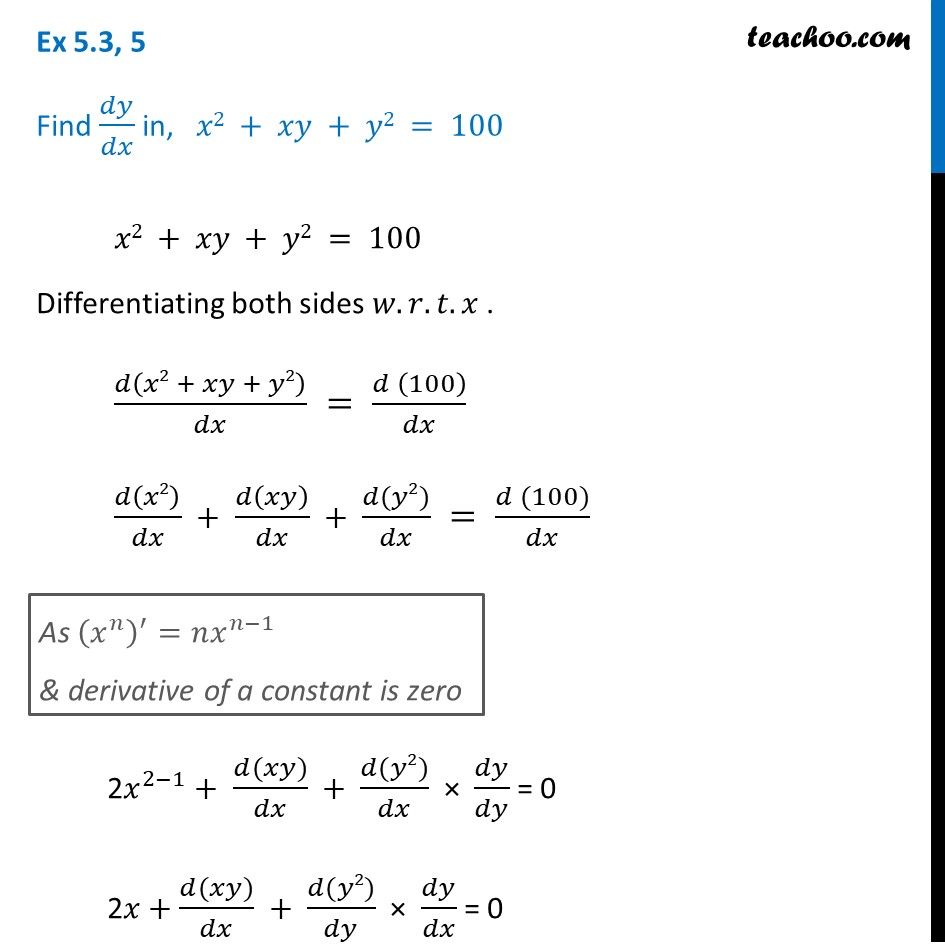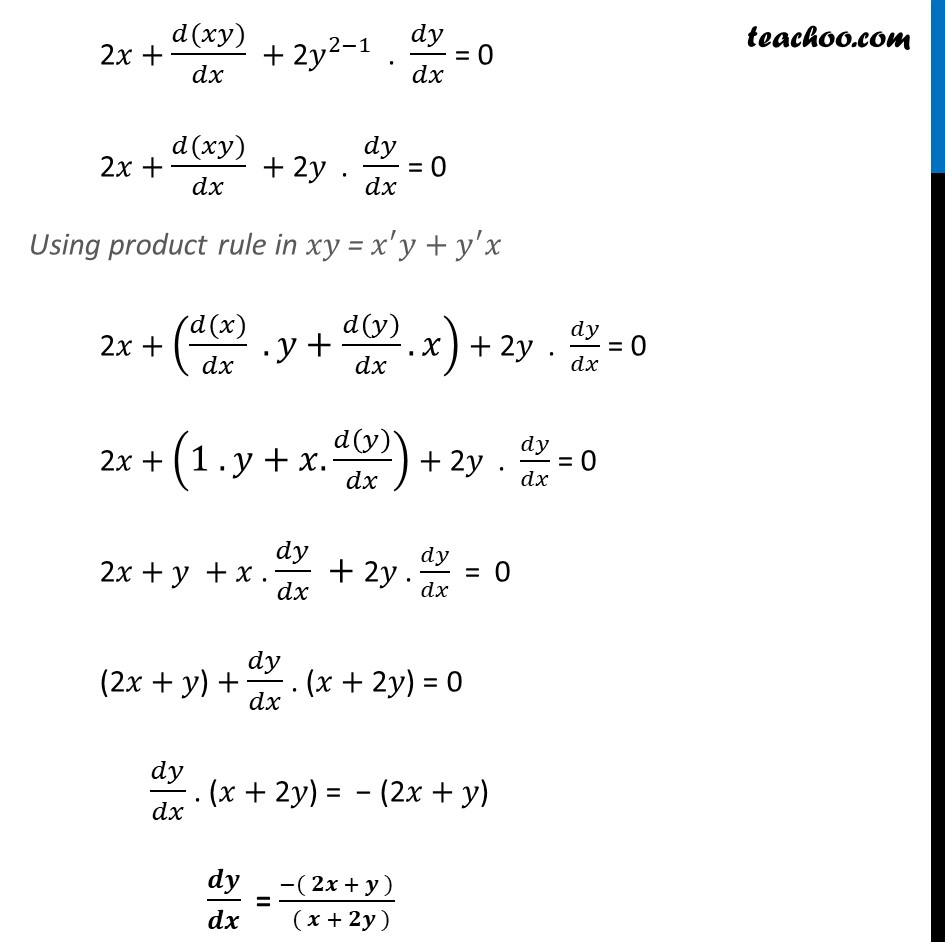Ex 5.3

Chapter 5 Class 12 Continuity and Differentiability
Serial order wiseLearn in your speed, with individual attention - Teachoo Maths 1-on-1 Class

### Transcript

Ex 5.3, 5 Find 𝑑𝑦/𝑑𝑥 in, 𝑥2 + 𝑥𝑦 + 𝑦2 = 100 𝑥2 + 𝑥𝑦 + 𝑦2 = 100 Differentiating both sides 𝑤.𝑟.𝑡.𝑥 . 𝑑(𝑥2 + 𝑥𝑦 + 𝑦2)/𝑑𝑥 = (𝑑 (100))/𝑑𝑥 𝑑(𝑥2)/𝑑𝑥 + 𝑑(𝑥𝑦)/𝑑𝑥 + (𝑑(𝑦2))/𝑑𝑥 = (𝑑 (100))/𝑑𝑥 2𝑥^(2−1) + (𝑑(𝑥𝑦))/𝑑𝑥 + (𝑑(𝑦2))/𝑑𝑥 × 𝑑𝑦/𝑑𝑦 = 0 2𝑥 + (𝑑(𝑥𝑦))/𝑑𝑥 + (𝑑(𝑦2))/𝑑𝑦 × 𝑑𝑦/𝑑𝑥 = 0 As (𝑥^𝑛 )^′=𝑛𝑥^(𝑛−1) & derivative of a constant is zero 2𝑥 + (𝑑(𝑥𝑦))/𝑑𝑥 + 2𝑦^(2−1) . 𝑑𝑦/𝑑𝑥 = 0 2𝑥 + (𝑑(𝑥𝑦))/𝑑𝑥 + 2𝑦 . 𝑑𝑦/𝑑𝑥 = 0 Using product rule in 𝑥𝑦 = 𝑥^′ 𝑦+𝑦^′ 𝑥 2𝑥 + ((𝑑(𝑥))/𝑑𝑥 .𝑦+𝑑(𝑦)/𝑑𝑥.𝑥) + 2𝑦 . 𝑑𝑦/𝑑𝑥 = 0 2𝑥 + (1 .𝑦+𝑥.𝑑(𝑦)/𝑑𝑥) + 2𝑦 . 𝑑𝑦/𝑑𝑥 = 0 2𝑥 + 𝑦 + 𝑥 . 𝑑𝑦/𝑑𝑥 + 2𝑦 . 𝑑𝑦/𝑑𝑥 = 0 (2𝑥 + 𝑦) + 𝑑𝑦/𝑑𝑥 . (𝑥 + 2𝑦) = 0 𝑑𝑦/𝑑𝑥 . (𝑥 + 2𝑦) = − (2𝑥 + 𝑦) 𝒅𝒚/𝒅𝒙 = (−( 𝟐𝒙 + 𝒚 ))/( ( 𝒙 + 𝟐𝒚 ))